Concept 77: Gordon (Constant) Growth Model and Multistage Dividend Discount Models | IFT World
101 Concepts for the Level I Exam

# Concept 77: Gordon (Constant) Growth Model and Multistage Dividend Discount ModelsGordon growth model (Constant growth dividend discount model): assumes that dividends will grow indefinitely at a constant growth rate. The value of the stock is calculated as: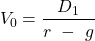Calculate the value of a stock that paid a $10 dividend last year, if dividends are expected to grow forever at 6% and the required rate of return on equity is 8%. Solution: D1 = D0 x (1 + dividend growth rate) =$10 x 1.06 = $10.6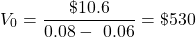g is the sustainable growth rate i.e. rate at which earnings and dividends can continue to grow indefinitely. It is calculated as: g = retention rate * ROE Multi-stage dividend discount model: used for companies with high growth rate over an initial few number of periods followed by a constant growth rate of dividends forever.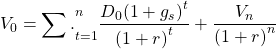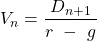Dividends of a company are expected to grow at 15% per year for three years, after which they are expected to grow at a constant rate of 5% per year. The last dividend paid was$2. Calculate the value of the stock of this company if the required rate of return is 10%.

Solution:

D1 = $2 x 1.15 =$2.3

D2 = $2.3 x 1.15 =$2.645

D3 = $2.645 x 1.15 =$3.042

D3 will grow at a constant growth rate of 5%. Hence,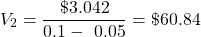Finally,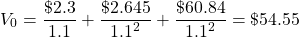Zoom Classes for Nov-23 Exam Starting Now!
This is default text for notification bar